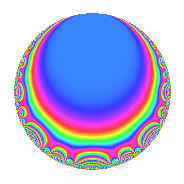# Properties

 Label 8008.2.a.iLevel 8008 Weight 2 Character orbit 8008.a Self dual Yes Analytic conductor 63.944 Analytic rank 1 Dimension 3 CM No Inner twists 1

# Related objects

## Newspace parameters

 Level: $$N$$ = $$8008 = 2^{3} \cdot 7 \cdot 11 \cdot 13$$ Weight: $$k$$ = $$2$$ Character orbit: $$[\chi]$$ = 8008.a (trivial)

## Newform invariants

 Self dual: Yes Analytic conductor: $$63.9442019386$$ Analytic rank: $$1$$ Dimension: $$3$$ Coefficient field: 3.3.229.1 Coefficient ring: $$\Z[a_1, a_2, a_3]$$ Coefficient ring index: $$1$$ Fricke sign: $$1$$ Sato-Tate group: $\mathrm{SU}(2)$

## $q$-expansion

Coefficients of the $$q$$-expansion are expressed in terms of a basis $$1,\beta_1,\beta_2$$ for the coefficient ring described below. We also show the integral $$q$$-expansion of the trace form.

 $$f(q)$$ $$=$$ $$q + ( 1 + \beta_{2} ) q^{3} + ( 2 - \beta_{1} ) q^{5} - q^{7} + ( 1 + \beta_{1} ) q^{9} +O(q^{10})$$ $$q + ( 1 + \beta_{2} ) q^{3} + ( 2 - \beta_{1} ) q^{5} - q^{7} + ( 1 + \beta_{1} ) q^{9} - q^{11} + q^{13} + ( 1 - 2 \beta_{1} + 2 \beta_{2} ) q^{15} + ( -5 + \beta_{1} - 2 \beta_{2} ) q^{17} + ( 2 \beta_{1} - \beta_{2} ) q^{19} + ( -1 - \beta_{2} ) q^{21} + ( -4 + 3 \beta_{1} + \beta_{2} ) q^{23} + ( 2 - 4 \beta_{1} + \beta_{2} ) q^{25} + ( -1 + 2 \beta_{1} - 2 \beta_{2} ) q^{27} + ( 2 - 2 \beta_{1} - 2 \beta_{2} ) q^{29} + ( -6 + \beta_{1} - \beta_{2} ) q^{31} + ( -1 - \beta_{2} ) q^{33} + ( -2 + \beta_{1} ) q^{35} + ( 2 + \beta_{1} - \beta_{2} ) q^{37} + ( 1 + \beta_{2} ) q^{39} + ( -2 - 6 \beta_{1} + 4 \beta_{2} ) q^{41} + ( 3 + 3 \beta_{1} ) q^{43} + ( -1 + \beta_{1} - \beta_{2} ) q^{45} + ( -4 \beta_{1} + 2 \beta_{2} ) q^{47} + q^{49} + ( -10 - 3 \beta_{2} ) q^{51} + ( -5 - \beta_{1} - 4 \beta_{2} ) q^{53} + ( -2 + \beta_{1} ) q^{55} + ( -1 + 3 \beta_{1} + \beta_{2} ) q^{57} + ( 2 + 3 \beta_{1} - 3 \beta_{2} ) q^{59} + ( 3 + \beta_{1} ) q^{61} + ( -1 - \beta_{1} ) q^{63} + ( 2 - \beta_{1} ) q^{65} + ( -3 - 2 \beta_{1} + \beta_{2} ) q^{67} + ( 2 + 7 \beta_{1} - 5 \beta_{2} ) q^{69} + ( -8 + \beta_{1} - 2 \beta_{2} ) q^{71} + ( -6 - 4 \beta_{1} ) q^{73} + ( 1 - 7 \beta_{1} + \beta_{2} ) q^{75} + q^{77} + ( 2 \beta_{1} + 3 \beta_{2} ) q^{79} + ( -8 - \beta_{1} + \beta_{2} ) q^{81} + ( 4 \beta_{1} + \beta_{2} ) q^{83} + ( -11 + 9 \beta_{1} - 5 \beta_{2} ) q^{85} + ( -6 - 6 \beta_{1} + 4 \beta_{2} ) q^{87} + ( 5 + 4 \beta_{1} - \beta_{2} ) q^{89} - q^{91} + ( -8 + \beta_{1} - 5 \beta_{2} ) q^{93} + ( -5 + 5 \beta_{1} - 4 \beta_{2} ) q^{95} + ( -2 + 5 \beta_{1} - 7 \beta_{2} ) q^{97} + ( -1 - \beta_{1} ) q^{99} +O(q^{100})$$ $$\operatorname{Tr}(f)(q)$$ $$=$$ $$3q + 2q^{3} + 6q^{5} - 3q^{7} + 3q^{9} + O(q^{10})$$ $$3q + 2q^{3} + 6q^{5} - 3q^{7} + 3q^{9} - 3q^{11} + 3q^{13} + q^{15} - 13q^{17} + q^{19} - 2q^{21} - 13q^{23} + 5q^{25} - q^{27} + 8q^{29} - 17q^{31} - 2q^{33} - 6q^{35} + 7q^{37} + 2q^{39} - 10q^{41} + 9q^{43} - 2q^{45} - 2q^{47} + 3q^{49} - 27q^{51} - 11q^{53} - 6q^{55} - 4q^{57} + 9q^{59} + 9q^{61} - 3q^{63} + 6q^{65} - 10q^{67} + 11q^{69} - 22q^{71} - 18q^{73} + 2q^{75} + 3q^{77} - 3q^{79} - 25q^{81} - q^{83} - 28q^{85} - 22q^{87} + 16q^{89} - 3q^{91} - 19q^{93} - 11q^{95} + q^{97} - 3q^{99} + O(q^{100})$$

Basis of coefficient ring in terms of a root $$\nu$$ of $$x^{3} - 4 x - 1$$:

 $$\beta_{0}$$ $$=$$ $$1$$ $$\beta_{1}$$ $$=$$ $$\nu$$ $$\beta_{2}$$ $$=$$ $$\nu^{2} - 3$$
 $$1$$ $$=$$ $$\beta_0$$ $$\nu$$ $$=$$ $$\beta_{1}$$ $$\nu^{2}$$ $$=$$ $$\beta_{2} + 3$$

## Embeddings

For each embedding $$\iota_m$$ of the coefficient field, the values $$\iota_m(a_n)$$ are shown below.

For more information on an embedded modular form you can click on its label.

Label $$\iota_m(\nu)$$ $$a_{2}$$ $$a_{3}$$ $$a_{4}$$ $$a_{5}$$ $$a_{6}$$ $$a_{7}$$ $$a_{8}$$ $$a_{9}$$ $$a_{10}$$
1.1
 −0.254102 −1.86081 2.11491
0 −1.93543 0 2.25410 0 −1.00000 0 0.745898 0
1.2 0 1.46260 0 3.86081 0 −1.00000 0 −0.860806 0
1.3 0 2.47283 0 −0.114908 0 −1.00000 0 3.11491 0
 $$n$$: e.g. 2-40 or 990-1000 Significant digits: Format: Complex embeddings Normalized embeddings Satake parameters Satake angles

## Inner twists

This newform does not admit any (nontrivial) inner twists.

## Atkin-Lehner signs

$$p$$ Sign
$$2$$ $$-1$$
$$7$$ $$1$$
$$11$$ $$1$$
$$13$$ $$-1$$

## Hecke kernels

This newform can be constructed as the intersection of the kernels of the following linear operators acting on $$S_{2}^{\mathrm{new}}(\Gamma_0(8008))$$:

 $$T_{3}^{3} - 2 T_{3}^{2} - 4 T_{3} + 7$$ $$T_{5}^{3} - 6 T_{5}^{2} + 8 T_{5} + 1$$ $$T_{17}^{3} + 13 T_{17}^{2} + 37 T_{17} - 28$$